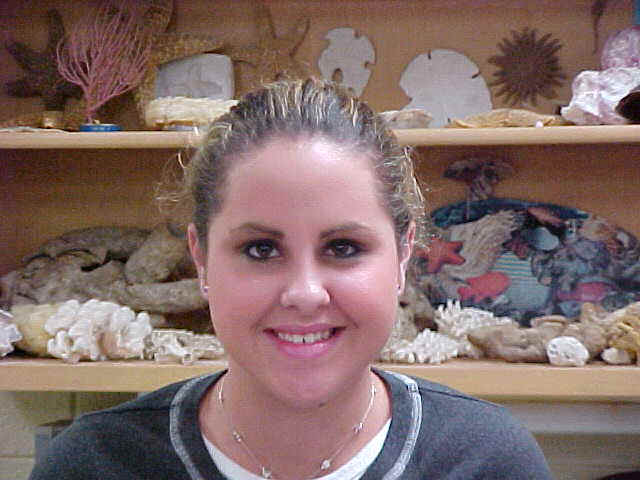# BI Sample 2 Lab Volume of Object

 Volume of an Irregular ObjectIntroduction

Everything is made of matter. Matter has physical and chemical properties. Physical properties are observable, such as mass, volume, and density. Mass is a fundamental property of an object generally regarded as equivalent to the amount of matter in the object. Volume is the amount of space it takes up. Density is the thickness of it the formula for density is D=M/V. The purpose of this experiment was to find the mass, volume, and density of three different objects.

Hypothesis

Determining the volume of an irregularly shaped object can help in determining density.

Materials

The materials used included a rubber stopper, a rock, a shell, a 100mL graduated cylinder, water, electronic balance, paper, and pencil.

Methods:

Obtain rubber a stopper, a shell, and a rock. Estimate and record the mass and volume of the three objects. Weigh and record each object. Take the graduated cylinder and fill halfway with water and record the volume. Add one object and record the new volume. Subtract initial volume from final, and record. Repeat with the other three objects.

Results:

 Object Estimated mass (g) Estimated volume (ml) Actual mass (g) Volume of H2O (ml) Volume of object + H2O (ml) Object’s Volume (ml) Density D=m/v(g/ml) Rubber Stopper 8g 65ml 8.3g 50ml 56ml 6ml 1.38g/ml Shell 2g 55ml 3.1g 50ml 53ml 3ml 1g/ml Rock 4g 60ml 7.8g 50ml 54ml 4ml 1.95g/ml

1. How did you determine the object’s:

a. Mass? Weighed it on a electronic balance

b. Volume? Put it in the water and measured the volume then subtracted the volume of the water before the object.

c. Density? Divided mass into volume

2. How did your estimates of mass and volume compare to the actual mass and volume of each object?

Our estimates of mass were close to the actual mass. Our estimates of volume were off not close to the actual volume at all.

3. Objects will sink if they are denser than water. Explain why ships made of steel float instead of sinking since steel is denser than water.

Because the ship has a hallow cavity with trapped air in it.

Error Analysis:

The volume of the water might not have been measured correctly.

Discussion and Conclusion:

The volume of the rubber stopper, rock and shell were determined by submerging them in a graduating cylinder containing water. The original water level in the cylinder was subtracted from the final water level to get the volume (ml) of each object. The actual mass (g) of each object was obtained by placing each on an electronic balance. Density could then be determined by dividing the mass of each object by its volume. The data showed the rock to have the greatest density at 1.95 g/ml with the rubber stopper 1.38 g/ml and the shell 1 g/ml being less dense.